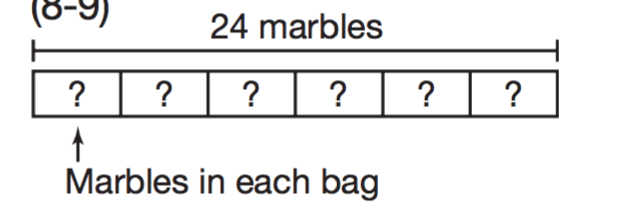Topic 8
Teacher's Name *
1. Which number makes both number sentences true? 7 X ■ = 42 42 ➗ 7 = ■ *
1 point
2. Which number sentence is true? *
1 point
3. Fred has 18 apples he wants to put equally into 3 baskets. Which number sentence is in the same fact family as 18 ➗ 3 = 6? *
1 point
4. Giselle has 40 tomato plants. She wants to put them into 5 rows with an equal number in each row. How many tomato plants will go in each row? *
1 point
5. There are 54 people going on a trip. They will travel in vans with 6 people in each van. How many vans are needed for all of the people? *
1 point
6. What number makes this equation true? n ➗ 8 = 6 *
1 point
7. Juan has 24 marbles and 6 bags. How many marbles he can put in each bag if he puts the same number of marbles in each? *
1 point8. Which number sentence is in the same fact family as 6 X 9 = 54? *
1 point
9. 27 divided by 9 is less than or greater than 32 divided by 8? *
1 point
10. Mario buys tickets to a play for a group. There are 2 adults and 4 children in the group. Adults’ tickets cost \$8 each. Children’s tickets cost \$5 each. How much does he pay for all the tickets? *
1 point